﻿ 混合直流异步联网系统运行特性研究 Research on the Operation Characteristics of Hybrid DC Asynchronous Interconnection System

Smart Grid
Vol. 08  No. 06 ( 2018 ), Article ID: 27764 , 11 pages
10.12677/SG.2018.86055

Research on the Operation Characteristics of Hybrid DC Asynchronous Interconnection System

Han Yan, Rui Song, Shichang Zhao, Xuan Wang, Chunlai Li, Jia Yang, Shaofei Wang

Power Research Institute of Qinghai Power Grid Company, Xining Qinghai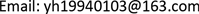Received: Nov. 6th, 2018; accepted: Nov. 21st, 2018; published: Nov. 28th, 2018ABSTRACT

Hybrid DC asynchronous interconnection system combines the advantages of LCC-HVDC and VSC-HVDC to provide a more flexible power grid interconnection mode. Firstly, this paper analyzes the circuit and control system of the LCC-HVDC and VSC-HVDC units. Then based on PSCAD/EMTDC simulation platform, a hybrid DC asynchronous interconnection simulation system is built to study the steady, dynamic, transient and fault recovery characteristics of hybrid DC asynchronous interconnection system. Finally, according to the research results, a measure to improve the voltage stability and fault recovery characteristics of LCC-HVDC system is proposed. The results show that hybrid DC asynchronous interconnection system has good steady-state and dynamic response. At the same time, hybrid DC asynchronous interconnection system can effectively improve the voltage support capability of the receiving grid by the excellent control characteristic of MMC-HVDC. More importantly, it can restrain the commutation failure of the LCC-HVDC station, improve the fault recovery characteristics of the AC/DC system and increase the reliability of the LCC-HVDC system.

Keywords:Hybrid DC Asynchronous Interconnection, LCC-HVDC, MMC-HVDC, Operation Characteristics, Commutation Failure, Fault Recovery1. 引言

2. 常规直流单元主回路及控制系统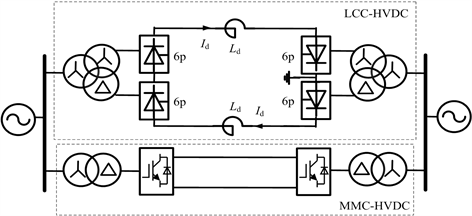Figure 1. Diagram of hybrid DC asynchronous interconnection system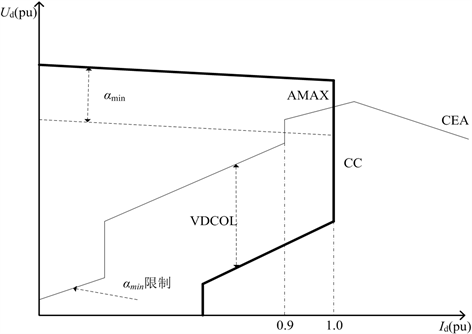Figure 2. Control characteristics of LCC-HVDC

3. 柔性直流单元主回路及控制系统

3.1. 内环控制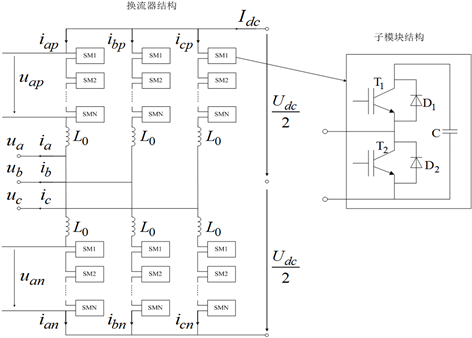Figure 3. Topology structure diagram of MMC-HVDC

$\left\{\begin{array}{l}{u}_{k}=\frac{{U}_{dc}}{2}-{e}_{kp}-{R}_{0}{i}_{kp}-{L}_{0}\frac{\text{d}{i}_{kp}}{\text{d}t}\\ {u}_{k}=-\frac{{U}_{dc}}{2}+{e}_{kn}+{R}_{0}{i}_{kn}+{L}_{0}\frac{\text{d}{i}_{kn}}{\text{d}t}\end{array}$ (1)

$\left\{\begin{array}{l}{i}_{kp}=\frac{{I}_{dc}}{3}+\frac{{i}_{k}}{2}\\ {i}_{kn}=\frac{{I}_{dc}}{3}-\frac{{i}_{k}}{2}\end{array}$ (2)

${e}_{k}={u}_{k}+\frac{1}{2}{R}_{0}{i}_{k}+\frac{1}{2}{L}_{0}\frac{\text{d}{i}_{k}}{\text{d}t}$ (3)

$\left\{\begin{array}{l}{e}_{d}={u}_{d}+\frac{1}{2}\left({R}_{0}+s{L}_{0}\right){i}_{d}+\frac{1}{2}\omega {L}_{0}{i}_{q}\\ {e}_{q}={u}_{q}+\frac{1}{2}\left({R}_{0}+s{L}_{0}\right){i}_{q}-\frac{1}{2}\omega {L}_{0}{i}_{d}\end{array}$ (4)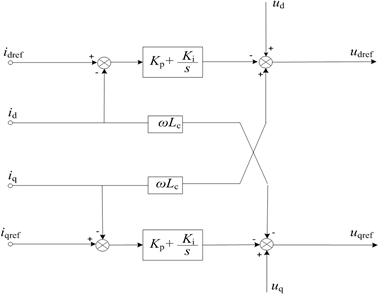Figure 4. Internal loop control block diagram of MMC

3.2. 外环控制Figure 5. Outer loop control block diagram of MMC

$\left\{\begin{array}{l}{e}_{d}^{+}={u}_{d}^{+}+\frac{1}{2}\left({R}_{0}+s{L}_{0}\right){i}_{d}^{+}+\frac{1}{2}\omega {L}_{0}{i}_{q}^{+}\\ {e}_{q}^{+}={u}_{q}^{+}+\frac{1}{2}\left({R}_{0}+s{L}_{0}\right){i}_{q}^{+}-\frac{1}{2}\omega {L}_{0}{i}_{d}^{+}\end{array}$ (5)

$\left\{\begin{array}{l}{e}_{d}^{-}={u}_{d}^{-}+\frac{1}{2}\left({R}_{0}+s{L}_{0}\right){i}_{d}^{-}+\frac{1}{2}\omega {L}_{0}{i}_{q}^{-}\\ {e}_{q}^{-}={u}_{q}^{-}+\frac{1}{2}\left({R}_{0}+s{L}_{0}\right){i}_{q}^{-}-\frac{1}{2}\omega {L}_{0}{i}_{d}^{-}\end{array}$ (6)

${u}_{k}={\text{e}}^{j\omega t}{u}_{dq}^{+}+{\text{e}}^{-j\omega t}{u}_{dq}^{-}$ (7)

$\left\{\begin{array}{l}{u}_{dq}^{+}={u}_{d}^{+}+j{u}_{q}^{+}\\ {u}_{dq}^{-}={u}_{d}^{-}+j{u}_{q}^{-}\end{array}$ (8)

${i}_{abc}={\text{e}}^{j\omega t}{i}_{dq}^{+}+{\text{e}}^{-j\omega t}{i}_{dq}^{-}$ (9)

$\left\{\begin{array}{l}{i}_{dq}^{+}={i}_{d}^{+}+j{i}_{q}^{+}\\ {i}_{dq}^{-}={i}_{d}^{-}+j{i}_{q}^{-}\end{array}$ (10)

$S=P+jQ=\left({\text{e}}^{j\omega t}{u}_{dq}^{+}+{\text{e}}^{-j\omega t}{u}_{dq}^{-}\right){\left({\text{e}}^{j\omega t}{i}_{dq}^{+}+{\text{e}}^{-j\omega t}{i}_{dq}^{-}\right)}^{*}$ (11)

$\left\{\begin{array}{l}P\left(t\right)={P}_{0}+{P}_{c2}\mathrm{cos}2\omega t+{P}_{s2}\mathrm{sin}2\omega t\\ Q\left(t\right)={Q}_{0}+{Q}_{c2}\mathrm{cos}2\omega t+{Q}_{s2}\mathrm{sin}2\omega t\end{array}$ (12)

$\left\{\begin{array}{l}{P}_{0}=1.5\left({u}_{d}^{+}{i}_{d}^{+}+{u}_{q}^{+}{i}_{q}^{+}+{u}_{d}^{-}{i}_{d}^{-}+{u}_{q}^{-}{i}_{q}^{-}\right)\\ {P}_{c2}=1.5\left({u}_{d}^{+}{i}_{d}^{-}+{u}_{q}^{+}{i}_{q}^{-}+{u}_{d}^{-}{i}_{d}^{+}+{u}_{q}^{-}{i}_{q}^{+}\right)\\ {P}_{s2}=1.5\left({u}_{q}^{-}{i}_{d}^{+}-{u}_{d}^{-}{i}_{q}^{+}-{u}_{q}^{+}{i}_{d}^{-}+{u}_{d}^{+}{i}_{q}^{-}\right)\\ {Q}_{0}=1.5\left({u}_{q}^{+}{i}_{d}^{+}-{u}_{d}^{+}{i}_{q}^{+}+{u}_{q}^{-}{i}_{d}^{-}-{u}_{d}^{-}{i}_{q}^{-}\right)\\ {Q}_{c2}=1.5\left({u}_{q}^{+}{i}_{d}^{-}-{u}_{d}^{+}{i}_{q}^{-}+{u}_{q}^{-}{i}_{d}^{+}-{u}_{d}^{-}{i}_{q}^{+}\right)\\ {Q}_{s2}=1.5\left({u}_{d}^{+}{i}_{d}^{-}+{u}_{q}^{+}{i}_{q}^{-}-{u}_{d}^{-}{i}_{d}^{+}-{u}_{q}^{-}{i}_{q}^{+}\right)\end{array}$ (13)

$\left\{\begin{array}{l}{P}_{0}=1.5{u}_{d}{i}_{d}\\ {Q}_{0}=-1.5{u}_{d}{i}_{q}\end{array}$ (14)

4. 混合直流异步联网系统运行特性研究

4.1. 稳态特性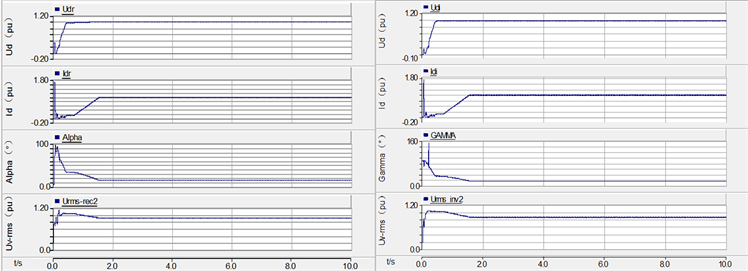(a) 常规直流单元整流侧 (b) 常规直流单元逆变侧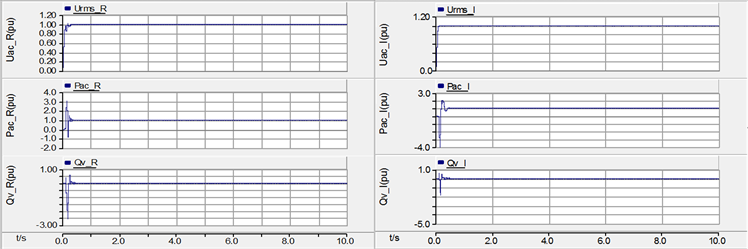(c) 柔性直流单元整流侧 (d) 柔性直流单元逆变侧

Figure 6. Steady-state operating curve of hybrid DC asynchronous interconnection system

4.2. 动态特性Figure 7. Current step response of LCC-HVDC

4.3. 暂态特性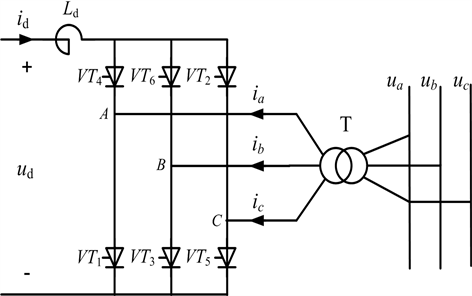Figure 8. Schematic of 6 pulse inverter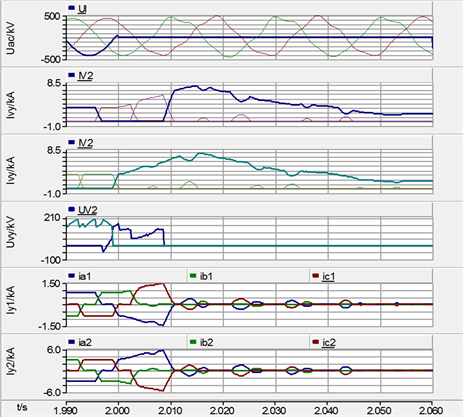(a) 常规直流单元单相接地故障波形(b) 柔性直流单元单相接地故障波形

Figure 9. Waveforms of hybrid DC asynchronous interconnection system when occurs single phase fault in AC system

5. 混合直流异步联网系统故障恢复特性研究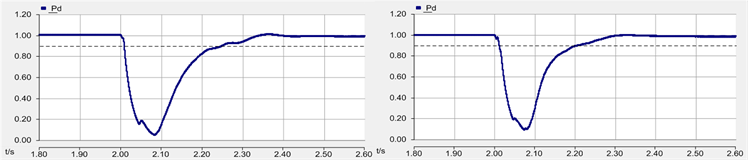(a) 柔性直流单元停运 (b) 柔性直流单元投运

Figure 10. DC transmission power waveforms of hybrid DC asynchronous interconnection system when occurs single phase fault in AC system

6. 结论

1) 混合直流异步联网系统创新性地采用常规直流单元与柔性直流单元并联结构，实现电网间异步联网，具有良好的稳态和动态特性，与工程实际相符；

2) 当受端电网故障时，常规直流单元发生换相失败，柔性直流单元能够起到STATCOM的作用，减小常规直流单元换相失败严重程度，提高常规直流输电运行可靠性，采用混合直流异步联网结构能够充分发挥柔性直流单元的优势；

3) 当混合直流异步联网系统馈入弱交流系统时，可以通过柔性直流单元无功控制改善常规直流单元电压稳定性及故障恢复特性。稳态时利用柔性直流单元与换流站无功补偿设备协调配合，优先采用柔性直流单元进行无功补偿，减少无功分组投切次数；暂态时利用柔性直流单元向常规直流单元提供动态无功支援，降低常规直流单元换相失败概率，改善常规直流单元故障恢复特性。

Research on the Operation Characteristics of Hybrid DC Asynchronous Interconnection System[J]. 智能电网, 2018, 08(06): 505-515. https://doi.org/10.12677/SG.2018.86055

1. 1. 赵婉君. 高压直流输电工程技术[M]. 第三版. 北京: 中国电力出版社, 2013: 7.

2. 2. 周保荣, 洪潮, 金小明, 等. 南方电网同步运行网架向异步运行网架的转变研究[J]. 中国电机工程学报, 2016, 36(8): 2084-2092.

3. 3. 徐政, 管敏渊. 柔性直流输电系统[M]. 北京: 机械工业出版社, 2013.

4. 4. 许冬. 混合多端直流输电运行特性研究[D]: [博士学位论文]. 北京: 华北电力大学(北京), 2017.

5. 5. 张东辉, 洪潮, 周保荣, 等. 云南电网与南方电网主网异步联网系统方案研究[J]. 南方电网技术, 2014, 8(6): 1-6.

6. 6. 雷霄, 王华伟, 曾南超, 等. LCC与VSC混联型多端高压直流输电系统运行特性的仿真研究[J]. 电工电能新技术, 2013, 32(2): 48-52.

7. 7. 姚文峰, 张勇, 洪潮, 等. 落点弱交流系统的直流输电系统运行特性及改善措施[J]. 南方电网技术, 2015, 9(4): 54-59.

8. 8. 张岩坡. 利用STATCOM改善直流输电系统运行特性的基础研究[D]: [硕士学位论文]. 保定: 华北电力大学, 2013.

9. 9. 汤广福, 罗湘, 魏晓光. 多端直流输电与直流电网技术[J]. 中国电机工程学报, 2013, 33(10): 8-17.

10. 10. 汤广福, 贺之渊, 庞辉. 柔性直流输电工程技术研究、应用及发展[J]. 电力系统自动化, 2013, 37(15): 3-14.

11. 11. 董云龙, 包海龙, 田杰, 等. 柔性直流输电控制及保护系统[J]. 电力系统自动化, 2011, 35(19): 89-92.

12. 12. 李兴源, 曾琦, 王渝红, 等. 柔性直流输电系统控制研究综述[J]. 高电压技术, 2016, 42(10): 3025-3037.

13. 13. 徐政, 刘高任, 张哲任. 柔性直流输电网的故障保护原理研究[J]. 高电压技术, 2017, 43(1): 1-8.

14. 14. 陈博怡, 李兴源, 胡永银, 等. 混合直流输电控制策略研究[J]. 高压电器, 2016(1): 118-123.

15. 15. 冯明, 李兴源, 李宽. 混合直流输电系统综述[J]. 现代电力, 2015, 32(2): 1-8.

16. 16. 许烽, 宣晓华, 江道灼, 等. 常规直流输电系统改造用的混合直流输电技术[J]. 电网技术, 2017, 41(10): 3209-3215.